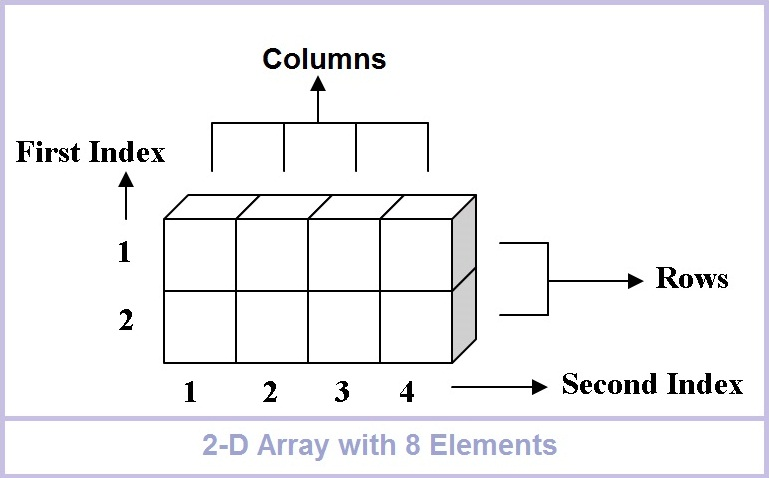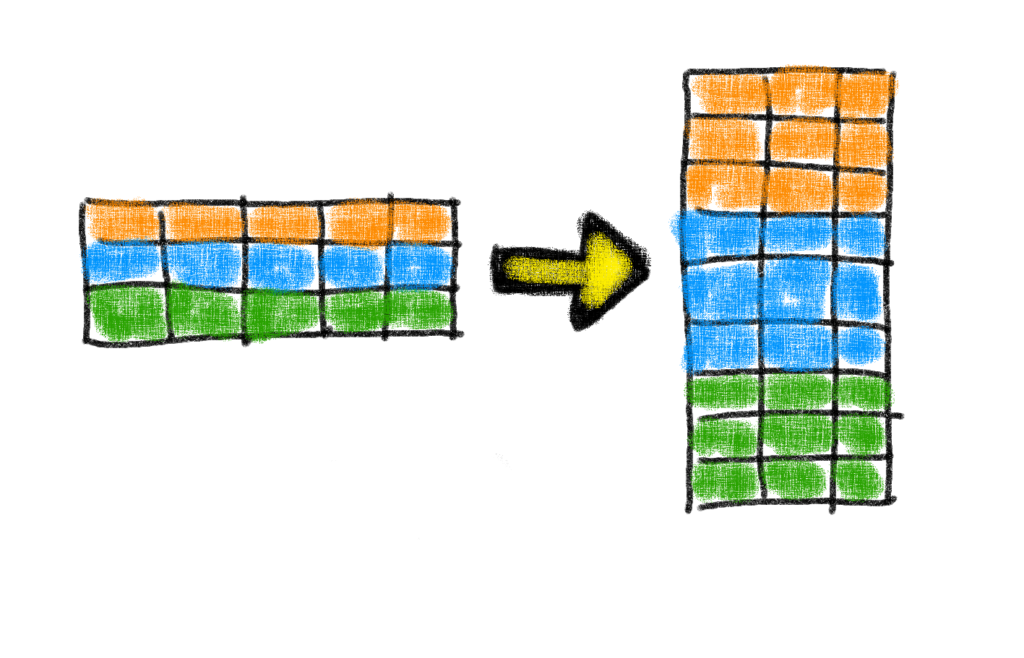# Data Transformation – Excel Array SolutionIn a recent Friday Challenge, I asked our readers to transform an varying text string into dates and values to determine averages.  Well although I was able to compete the challenge, that wasn’t good enough.  Now lets look at an Excel Array solution for the same problem that is more efficient than copying the other formulas down 10,000 rows.

Here is the original challenge: Friday Challenge – Data Transformation

You can read about the original solution using formulas that will be used in this solution but put into an Excel Array formula: Friday Challenge – Data Transformation Solution## The Problem

Transform the previous non-array formula solution into an Array formula.

## The Breakdown

We will use our existing formulas for the KPBS and the Date and combine them into an Array Formula.

First, we will create one Array formula for the KPBS total average.

Second, we will create one Array formula for the KPBS average for the month of May.## Excel Array Solution

In the previous challenge linked above, we created formulas to breakdown the text string into KBPS and Date.

Here is a sample set of data and formulas used in the solution:

A B C
1 Sample Data Date Solution KPBS Solution
2 __11 AM   27-Mar-2016___4.970 kbps 3/27/2016 4.97
Worksheet Formulas
Cell Formula
B2 =IFERROR(VALUE(MID(A2,FIND(“-“,A2)-2,11)),VALUE(MID(A2,FIND(“-“,A2)-4,2)&MID(A2,FIND(“-“,A2),9)))
C2 =VALUE(SUBSTITUTE(MID(A2,FIND(2016,A2)+4,FIND(“kbps”,A2)-FIND(2016,A2)-4),”_”,””))

So how can we transform these formulas into Excel Array formulas?  Lets go back to the original challenge for each question.

### Question 1:

The original challenge was to find the KBPS average of the entire data set.  So to do that, we would start with the formula in cell C2.

This formula finds the value of an individual cell.  In order to have it calculate on the range, we need to change the formula references to ranges.  Therefore, the first change is to modify the cell reference of A2 to a range of cells A2:A10001.  However, if we make this an array formula, we will not get the answer desired as it just displays a value.  Excel cannot display 10,000 values in one spreadsheet cell.  So we need to aggregate the values with another function.

The request was for an Average, so we will use that Excel Function to Aggregate the 10,000 values.

So simply wrap the formula in an Average function and press CTRL+SHIFT+ENTER and you will find our answer.  Your final formula will look like this:

Average 5.042867
Cell Formula
F3 {=AVERAGE(VALUE(SUBSTITUTE(MID(A2:A10001,FIND(2016,A2:A10001)+4,FIND(“kbps”,A2:A10001)-FIND(2016,A2:A10001)-4),”_”,””)))}

Entered with Ctrl+Shift+Enter. If entered correctly, Excel will surround with curly braces {}.
Note: Do not try and enter the {} manually yourself

### Question 2:

The original challenge also wanted us to find the KBPS average for the Month of May.  So to do that, we would start with the formula in cell B2.

# If you found the website and tutorials helpful, please consider donating to keep the lights on.

## Donate with PayPal here:Now the formula in cell B2 is just a date.  But we want to check and see if that date = May.  If we wrap that formula in a MONTH function that is how we can check if it equals May, as May=5 in Excel terms.

Since we want to check and see if it equals may, we should wrap that in a MONTH Function and see if it equals 5 (the month of May = 5 in Excel terms).  Then wrap it in an IF statement to check the condition.  That formula would look like this:

=IF(MONTH(IFERROR(VALUE(MID(A2,FIND(“-“,A2)-2,11)),VALUE(MID(A2,FIND(“-“,A2)-4,2)&MID(A2,FIND(“-“,A2),9))))=5,))

But this is just an IF function, what if it is true?  If it is true, then we want to return all the KBPS values so that we can average them.  We created that formula in Question 1 above.  So we would want to modify our formula by adding that as our TRUE value for the Excel IF function.  Then our formula would look like this:

=IF(MONTH(IFERROR(VALUE(MID(A2,FIND(“-“,A2)-2,11)),VALUE(MID(A2,FIND(“-“,A2)-4,2)&MID(A2,FIND(“-“,A2),9))))=5,VALUE(SUBSTITUTE(MID(A2:A10001,FIND(2016,A2:A10001)+4,FIND(“kbps”,A2:A10001)-FIND(2016,A2:A10001)-4),”_”,””)))

However, our original Date formula doesn’t have a range, but only a cell reference of A2.  So Find and Replace A2 with A2:A10001.  Your formula would then look like this:

=IF(MONTH(IFERROR(VALUE(MID(A2:A10001,FIND(“-“,A2:A10001)-2,11)),VALUE(MID(A2:A10001,FIND(“-“,A2:A10001)-4,2)&MID(A2:A10001,FIND(“-“,A2:A10001),9))))=5,VALUE(SUBSTITUTE(MID(A2:A10001:A10001,FIND(2016,A2:A10001:A10001)+4,FIND(“kbps”,A2:A10001:A10001)-FIND(2016,A2:A10001:A10001)-4),”_”,””)))

Now that we our formula worked out to return a range of KPBS values if its matching date equals may, we just need to find the average of that range of values.  To do that, just wrap the formula in an Average Function and then complete it by pressing CTRL+SHIFT+ENTER to make it an Excel Array Formula.  You can see the final formula below.

May Avg 5.181021
Array Formulas
Cell Formula
F2 {=AVERAGE(IF(MONTH(IFERROR(VALUE(MID(A2:A10001,FIND(“-“,A2:A10001)-2,11)),VALUE(MID(A2:A10001,FIND(“-“,A2:A10001)-4,2)&MID(A2:A10001,FIND(“-“,A2:A10001),9))))=5,VALUE(SUBSTITUTE(MID(A2:A10001,FIND(2016,A2:A10001)+4,FIND(“kbps”,A2:A10001)-FIND(2016,A2:A10001)-4),”_”,””))))}

Entered with Ctrl+Shift+Enter. If entered correctly, Excel will surround with curly braces {}.
Note: Do not try and enter the {} manually yourself

## Conclusion

With an Excel Array formula, we don’t need to copy the formulas down for every row.  That will save space on the server.  Array formulas are so powerful and not too complicated once you learn the mechanics.  I say give it a try when you have many conditions to check.  First create your base formulas and then imbed them in Array to make it easier.

You can see the array formula in action with this free download file: Data-Transformation-Solution-Array.xlsx

## Video Demonstration

Also, thanks to “Hocine Satour” for his array formula that inspired this post.

Are you afraid of Excel Array formulas or do you love them?  Let me know in the comments below.

Don’t forget to subscribe to our newsletter so that you get the next post in your inbox below.

Steve=True